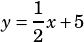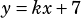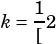Hi Mike… QAS March 2021, Section 3, # 20. Since “no solution,” can you consider each side a separate parallel line & use corresponding coefficients to confirm that k must equal 1/2? Or, what’s the best way to solve?

1/2x + 5 = kx + 7

In the given equation, k is a constant. The equation has no solution. What is the value of k?

Yes, the way you describe is perfect and exactly how I’d go about it! No solutions means the linesandwill never intersect, which means they must have the same slope! So you know/